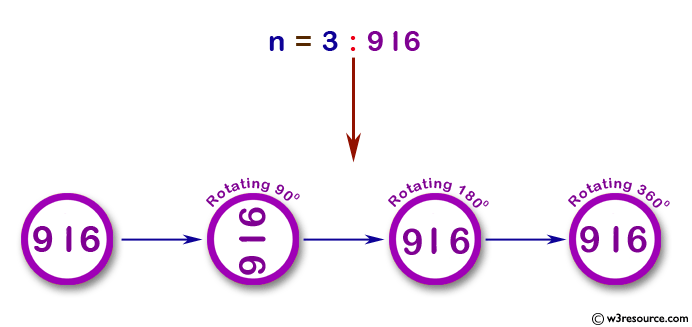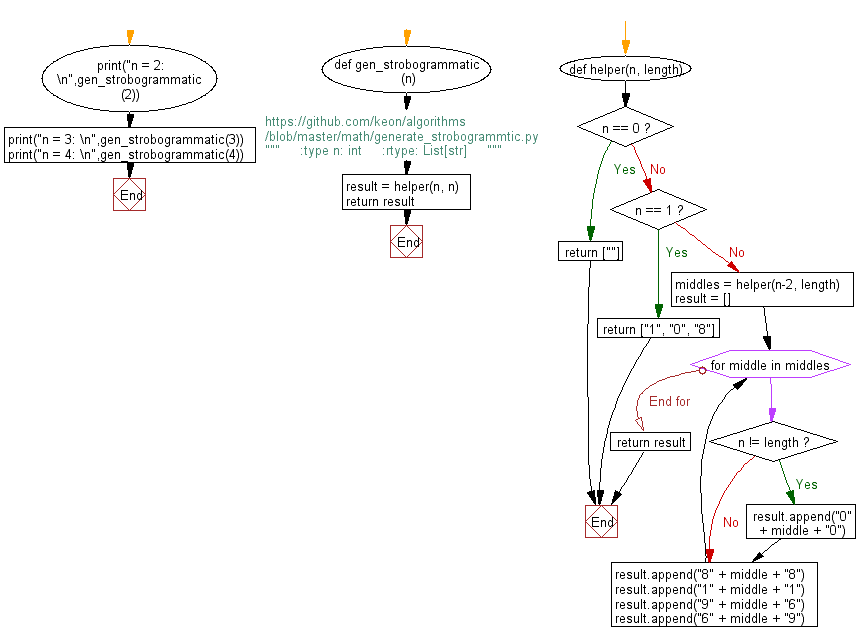﻿ Python: Get all strobogrammatic numbers that are of length n - w3resource# Python: Get all strobogrammatic numbers that are of length n

## Python Basic - 1: Exercise-17 with Solution

Write a Python program to get all strobogrammatic numbers that are of length n.

A strobogrammatic number is a number whose numeral is rotationally symmetric, so that it appears the same when rotated 180 degrees. In other words, the numeral looks the same right-side up and upside down (e.g., 69, 96, 1001).

For example,
Given n = 2, return ["11", "69", "88", "96"].
Given n = 3, return ['818', '111', '916', '619', '808', '101', '906', '609', '888', '181', '986', '689']

Pictorial Presentation:Sample Solution:

Python Code:

``````#https://github.com/keon/algorithms/blob/master/math/generate_strobogrammtic.py
def gen_strobogrammatic(n):
"""
:type n: int
:rtype: List[str]
"""
result = helper(n, n)
return result

def helper(n, length):
if n == 0:
return [""]
if n == 1:
return ["1", "0", "8"]
middles = helper(n-2, length)
result = []
for middle in middles:
if n != length:
result.append("0" + middle + "0")
result.append("8" + middle + "8")
result.append("1" + middle + "1")
result.append("9" + middle + "6")
result.append("6" + middle + "9")
return result

print("n = 2: \n",gen_strobogrammatic(2))
print("n = 3: \n",gen_strobogrammatic(3))
print("n = 4: \n",gen_strobogrammatic(4))
``````

Sample Output:

```n = 2:
['88', '11', '96', '69']
n = 3:
['818', '111', '916', '619', '808', '101', '906', '609', '888', '181', '986', '689']
n = 4:
['8008', '1001', '9006', '6009', '8888', '1881', '9886', '6889', '8118', '1111', '9116', '6119', '8968', '1961', '9966', '6969', '8698', '1691', '9696', '6699']
```

Flowchart:## Visualize Python code execution:

The following tool visualize what the computer is doing step-by-step as it executes the said program:

Python Code Editor :

Have another way to solve this solution? Contribute your code (and comments) through Disqus.

What is the difficulty level of this exercise?

Test your Programming skills with w3resource's quiz.

﻿

## Python: Tips of the Day

What is the difference between Python's list methods append and extend?

append: Appends object at the end.

```x = [1, 2, 3]
x.append([4, 5])
print (x)
```

Output:

```[1, 2, 3, [4, 5]]
```

extend: Extends list by appending elements from the iterable.

```x = [1, 2, 3]
x.extend([4, 5])
print (x)
```

Output:

```[1, 2, 3, 4, 5]
```

Ref: https://bit.ly/2AZ6ZFq